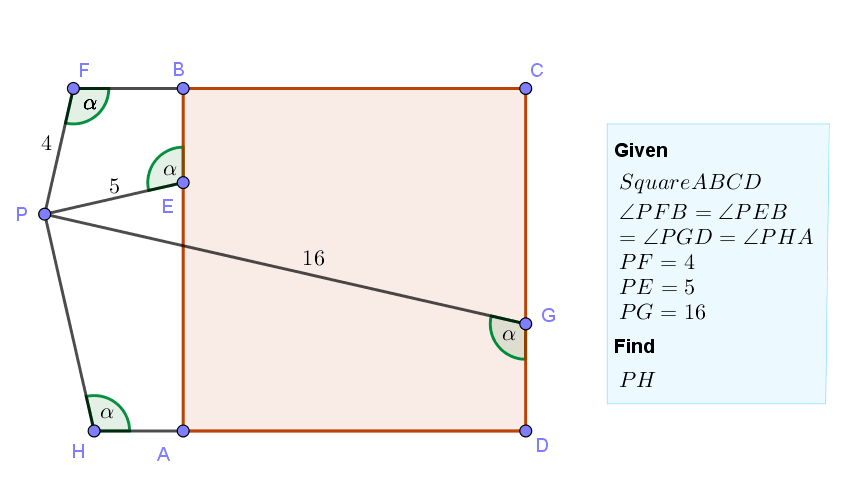# Geometry Problem 1501: Square, Exterior Point, Congruent Angles, Segment, Measurement

Given square ABCD with $$\angle PFB=\angle PEB=\angle PGD=\angle PHA=\alpha$$ and $$PF=4,PE=5,PG=16$$. Find $$PH$$.